Hypothesis Testing with One Sample

# 46 Distribution Needed for Hypothesis Testing

Earlier, we discussed sampling distributions. Particular distributions are associated with hypothesis testing.We will perform hypotheses tests of a population mean using a normal distribution or a Student’s t-distribution. (Remember, use a Student’s t-distribution when the population standard deviation is unknown and the sample size is small, where small is considered to be less than 30 observations.) We perform tests of a population proportion using a normal distribution when we can assume that the distribution is normally distributed. We consider this to be true if the sample proportion,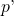, times the sample size is greater than 5 and 1-times the sample size is also greater then 5. This is the same rule of thumb we used when developing the formula for the confidence interval for a population proportion.

### Hypothesis Test for the Mean

Going back to the standardizing formula we can derive the test statistic for testing hypotheses concerning means.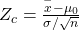The standardizing formula can not be solved as it is because we do not have μ, the population mean. However, if we substitute in the hypothesized value of the mean, μ0 in the formula as above, we can compute a Z value. This is the test statistic for a test of hypothesis for a mean and is presented in (Figure). We interpret this Z value as the associated probability that a sample with a sample mean of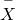could have come from a distribution with a population mean of H0 and we call this Z value Zc for “calculated”. (Figure) and (Figure) show this process.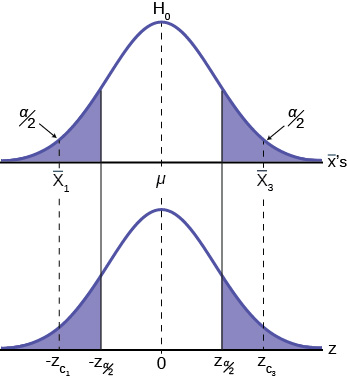In (Figure) two of the three possible outcomes are presented.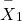and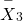are in the tails of the hypothesized distribution of H0. Notice that the horizontal axis in the top panel is labeled‘s. This is the same theoretical distribution of‘s, the sampling distribution, that the Central Limit Theorem tells us is normally distributed. This is why we can draw it with this shape. The horizontal axis of the bottom panel is labeled Z and is the standard normal distribution.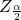and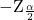, called the critical values, are marked on the bottom panel as the Z values associated with the probability the analyst has set as the level of significance in the test, (α). The probabilities in the tails of both panels are, therefore, the same.

Notice that for eachthere is an associated Zc, called the calculated Z, that comes from solving the equation above. This calculated Z is nothing more than the number of standard deviations that the hypothesized mean is from the sample mean. If the sample mean falls “too many” standard deviations from the hypothesized mean we conclude that the sample mean could not have come from the distribution with the hypothesized mean, given our pre-set required level of significance. It could have come from H0, but it is deemed just too unlikely. In (Figure) both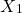and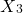are in the tails of the distribution. They are deemed “too far” from the hypothesized value of the mean given the chosen level of alpha. If in fact this sample mean it did come from H0, but from in the tail, we have made a Type I error: we have rejected a good null. Our only real comfort is that we know the probability of making such an error, α, and we can control the size of α.

(Figure) shows the third possibility for the location of the sample mean,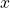. Here the sample mean is within the two critical values. That is, within the probability of (1-α) and we cannot reject the null hypothesis.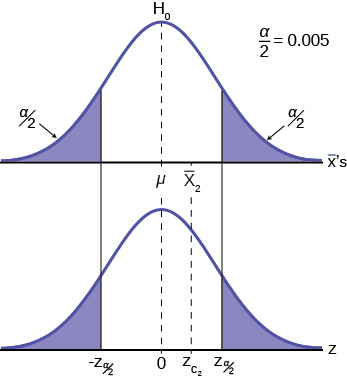This gives us the decision rule for testing a hypothesis for a two-tailed test:

Decision rule: two-tail test
If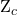<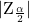: then cannot REJECT H0
If>: then cannot ACCEPT H0

This rule will always be the same no matter what hypothesis we are testing or what formulas we are using to make the test. The only change will be to change the Zc to the appropriate symbol for the test statistic for the parameter being tested. Stating the decision rule another way: if the sample mean is unlikely to have come from the distribution with the hypothesized mean we cannot accept the null hypothesis. Here we define “unlikely” as having a probability less than alpha of occurring.

### P-Value Approach

An alternative decision rule can be developed by calculating the probability that a sample mean could be found that would give a test statistic larger than the test statistic found from the current sample data assuming that the null hypothesis is true. Here the notion of “likely” and “unlikely” is defined by the probability of drawing a sample with a mean from a population with the hypothesized mean that is either larger or smaller than that found in the sample data. Simply stated, the p-value approach compares the desired significance level, α, to the p-value which is the probability of drawing a sample mean further from the hypothesized value than the actual sample mean. A large p-value calculated from the data indicates that we should not reject the null hypothesis. The smaller the p-value, the more unlikely the outcome, and the stronger the evidence is against the null hypothesis. We would reject the null hypothesis if the evidence is strongly against it. The relationship between the decision rule of comparing the calculated test statistics, Zc, and the Critical Value, Zα , and using the p-value can be seen in (Figure).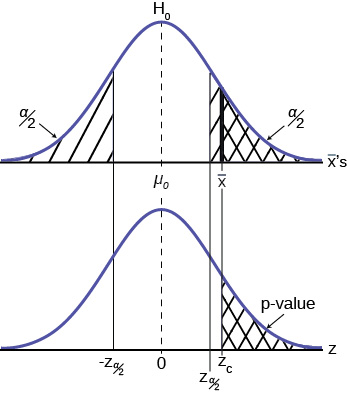The calculated value of the test statistic is Zc in this example and is marked on the bottom graph of the standard normal distribution because it is a Z value. In this case the calculated value is in the tail and thus we cannot accept the null hypothesis, the associatedis just too unusually large to believe that it came from the distribution with a mean of µ0 with a significance level of α.

If we use the p-value decision rule we need one more step. We need to find in the standard normal table the probability associated with the calculated test statistic, Zc. We then compare that to the α associated with our selected level of confidence. In (Figure) we see that the p-value is less than α and therefore we cannot accept the null. We know that the p-value is less than α because the area under the p-value is smaller than α/2. It is important to note that two researchers drawing randomly from the same population may find two different P-values from their samples. This occurs because the P-value is calculated as the probability in the tail beyond the sample mean assuming that the null hypothesis is correct. Because the sample means will in all likelihood be different this will create two different P-values. Nevertheless, the conclusions as to the null hypothesis should be different with only the level of probability of α.

Here is a systematic way to make a decision of whether you cannot accept or cannot reject a null hypothesis if using the p-value and a preset or preconceived α (the “significance level“). A preset α is the probability of a Type I error (rejecting the null hypothesis when the null hypothesis is true). It may or may not be given to you at the beginning of the problem. In any case, the value of α is the decision of the analyst. When you make a decision to reject or not reject H0, do as follows:

• If α > p-value, cannot accept H0. The results of the sample data are significant. There is sufficient evidence to conclude that H0 is an incorrect belief and that the alternative hypothesis, Ha, may be correct.
• If α ≤ p-value, cannot reject H0. The results of the sample data are not significant. There is not sufficient evidence to conclude that the alternative hypothesis, Ha, may be correct. In this case the status quo stands.
• When you “cannot reject H0“, it does not mean that you should believe that H0 is true. It simply means that the sample data have failed to provide sufficient evidence to cast serious doubt about the truthfulness of H0. Remember that the null is the status quo and it takes high probability to overthrow the status quo. This bias in favor of the null hypothesis is what gives rise to the statement “tyranny of the status quo” when discussing hypothesis testing and the scientific method.

Both decision rules will result in the same decision and it is a matter of preference which one is used.

### One and Two-tailed Tests

The discussion of (Figure)(Figure) was based on the null and alternative hypothesis presented in (Figure). This was called a two-tailed test because the alternative hypothesis allowed that the mean could have come from a population which was either larger or smaller than the hypothesized mean in the null hypothesis. This could be seen by the statement of the alternative hypothesis as μ ≠ 100, in this example.

It may be that the analyst has no concern about the value being “too” high or “too” low from the hypothesized value. If this is the case, it becomes a one-tailed test and all of the alpha probability is placed in just one tail and not split into α/2 as in the above case of a two-tailed test. Any test of a claim will be a one-tailed test. For example, a car manufacturer claims that their Model 17B provides gas mileage of greater than 25 miles per gallon. The null and alternative hypothesis would be:

• H0: µ ≤ 25
• Ha: µ > 25

The claim would be in the alternative hypothesis. The burden of proof in hypothesis testing is carried in the alternative. This is because failing to reject the null, the status quo, must be accomplished with 90 or 95 percent significance that it cannot be maintained. Said another way, we want to have only a 5 or 10 percent probability of making a Type I error, rejecting a good null; overthrowing the status quo.

This is a one-tailed test and all of the alpha probability is placed in just one tail and not split into α/2 as in the above case of a two-tailed test.

(Figure) shows the two possible cases and the form of the null and alternative hypothesis that give rise to them.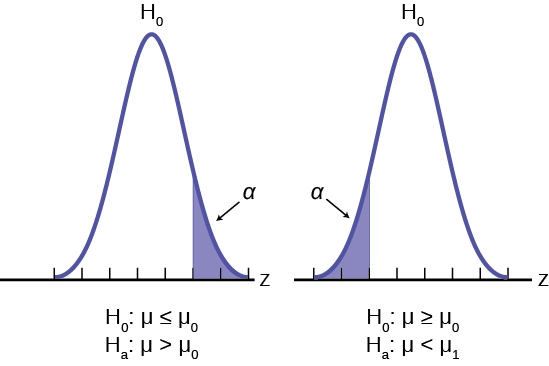where μ0 is the hypothesized value of the population mean.

Test Statistics for Test of Means, Varying Sample Size, Population Standard Deviation Known or Unknown
Sample size Test statistic
< 30
(σ unknown)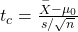< 30
(σ known)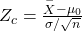> 30
(σ unknown)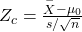> 30
(σ known)### Effects of Sample Size on Test Statistic

In developing the confidence intervals for the mean from a sample, we found that most often we would not have the population standard deviation, σ. If the sample size were larger than 30, we could simply substitute the point estimate for σ, the sample standard deviation, s, and use the student’s t distribution to correct for this lack of information.

When testing hypotheses we are faced with this same problem and the solution is exactly the same. Namely: If the population standard deviation is unknown, and the sample size is less than 30, substitute s, the point estimate for the population standard deviation, σ, in the formula for the test statistic and use the student’s t distribution. All the formulas and figures above are unchanged except for this substitution and changing the Z distribution to the student’s t distribution on the graph. Remember that the student’s t distribution can only be computed knowing the proper degrees of freedom for the problem. In this case, the degrees of freedom is computed as before with confidence intervals: df = (n-1). The calculated t-value is compared to the t-value associated with the pre-set level of confidence required in the test, tα, df found in the student’s t tables. If we do not know σ, but the sample size is 30 or more, we simply substitute s for σ and use the normal distribution.

(Figure) summarizes these rules.

### A Systematic Approach for Testing A Hypothesis

A systematic approach to hypothesis testing follows the following steps and in this order. This template will work for all hypotheses that you will ever test.

• Set up the null and alternative hypothesis. This is typically the hardest part of the process. Here the question being asked is reviewed. What parameter is being tested, a mean, a proportion, differences in means, etc. Is this a one-tailed test or two-tailed test? Remember, if someone is making a claim it will always be a one-tailed test.
• Decide the level of significance required for this particular case and determine the critical value. These can be found in the appropriate statistical table. The levels of confidence typical for the social sciences are 90, 95 and 99. However, the level of significance is a policy decision and should be based upon the risk of making a Type I error, rejecting a good null. Consider the consequences of making a Type I error.

Next, on the basis of the hypotheses and sample size, select the appropriate test statistic and find the relevant critical value: Zα, tα, etc. Drawing the relevant probability distribution and marking the critical value is always big help. Be sure to match the graph with the hypothesis, especially if it is a one-tailed test.

• Take a sample(s) and calculate the relevant parameters: sample mean, standard deviation, or proportion. Using the formula for the test statistic from above in step 2, now calculate the test statistic for this particular case using the parameters you have just calculated.
• Compare the calculated test statistic and the critical value. Marking these on the graph will give a good visual picture of the situation. There are now only two situations:
1. The test statistic is in the tail: Cannot Accept the null, the probability that this sample mean (proportion) came from the hypothesized distribution is too small to believe that it is the real home of these sample data.
2. The test statistic is not in the tail: Cannot Reject the null, the sample data are compatible with the hypothesized population parameter.
• Reach a conclusion. It is best to articulate the conclusion two different ways. First a formal statistical conclusion such as “With a 95 % level of significance we cannot accept the null hypotheses that the population mean is equal to XX (units of measurement)”. The second statement of the conclusion is less formal and states the action, or lack of action, required. If the formal conclusion was that above, then the informal one might be, “The machine is broken and we need to shut it down and call for repairs”.

All hypotheses tested will go through this same process. The only changes are the relevant formulas and those are determined by the hypothesis required to answer the original question.

### Chapter Review

In order for a hypothesis test’s results to be generalized to a population, certain requirements must be satisfied.

When testing for a single population mean:

1. A Student’s t-test should be used if the data come from a simple, random sample and the population is approximately normally distributed, or the sample size is large, with an unknown standard deviation.
2. The normal test will work if the data come from a simple, random sample and the population is approximately normally distributed, or the sample size is large.

When testing a single population proportion use a normal test for a single population proportion if the data comes from a simple, random sample, fill the requirements for a binomial distribution, and the mean number of success and the mean number of failures satisfy the conditions: np > 5 and nq > n where n is the sample size, p is the probability of a success, and q is the probability of a failure.

### Formula Review

Test Statistics for Test of Means, Varying Sample Size, Population Known or Unknown
Sample size Test statistic
< 30
(σ unknown)< 30
(σ known)> 30
(σ unknown)> 30
(σ known)Which two distributions can you use for hypothesis testing for this chapter?

A normal distribution or a Student’s t-distribution

Which distribution do you use when you are testing a population mean and the population standard deviation is known? Assume sample size is large. Assume a normal distribution with n ≥ 30.

Which distribution do you use when the standard deviation is not known and you are testing one population mean? Assume a normal distribution, with n ≥ 30.

Use a Student’s t-distribution

A population mean is 13. The sample mean is 12.8, and the sample standard deviation is two. The sample size is 20. What distribution should you use to perform a hypothesis test? Assume the underlying population is normal.

A population has a mean is 25 and a standard deviation of five. The sample mean is 24, and the sample size is 108. What distribution should you use to perform a hypothesis test?

a normal distribution for a single population mean

It is thought that 42% of respondents in a taste test would prefer Brand A. In a particular test of 100 people, 39% preferred Brand A. What distribution should you use to perform a hypothesis test?

You are performing a hypothesis test of a single population mean using a Student’s t-distribution. What must you assume about the distribution of the data?

It must be approximately normally distributed.

You are performing a hypothesis test of a single population mean using a Student’s t-distribution. The data are not from a simple random sample. Can you accurately perform the hypothesis test?

You are performing a hypothesis test of a single population proportion. What must be true about the quantities of np and nq?

They must both be greater than five.

You are performing a hypothesis test of a single population proportion. You find out that np is less than five. What must you do to be able to perform a valid hypothesis test?

You are performing a hypothesis test of a single population proportion. The data come from which distribution?

binomial distribution

### Homework

It is believed that Lake Tahoe Community College (LTCC) Intermediate Algebra students get less than seven hours of sleep per night, on average. A survey of 22 LTCC Intermediate Algebra students generated a mean of 7.24 hours with a standard deviation of 1.93 hours. At a level of significance of 5%, do LTCC Intermediate Algebra students get less than seven hours of sleep per night, on average? The distribution to be used for this test is~ ________________

1.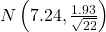2.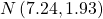3. t22
4. t21

d

### Key Terms

Binomial Distribution
a discrete random variable (RV) that arises from Bernoulli trials. There are a fixed number, n, of independent trials. “Independent” means that the result of any trial (for example, trial 1) does not affect the results of the following trials, and all trials are conducted under the same conditions. Under these circumstances the binomial RV Χ is defined as the number of successes in n trials. The notation is: X ~ B(n, p) μ = np and the standard deviation is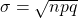. The probability of exactly x successes in n trials is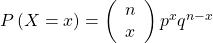.
Normal Distribution
a continuous random variable (RV) with pdf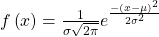, where μ is the mean of the distribution, and σ is the standard deviation, notation: X ~ N(μ, σ). If μ = 0 and σ = 1, the RV is called the standard normal distribution.
Standard Deviation
a number that is equal to the square root of the variance and measures how far data values are from their mean; notation: s for sample standard deviation and σ for population standard deviation.
Student’s t-Distribution
investigated and reported by William S. Gossett in 1908 and published under the pseudonym Student. The major characteristics of the random variable (RV) are:

• It is continuous and assumes any real values.
• The pdf is symmetrical about its mean of zero. However, it is more spread out and flatter at the apex than the normal distribution.
• It approaches the standard normal distribution as n gets larger.
• There is a “family” of t distributions: every representative of the family is completely defined by the number of degrees of freedom which is one less than the number of data items.
Test Statistic
The formula that counts the number of standard deviations on the relevant distribution that estimated parameter is away from the hypothesized value.
Critical Value
The t or Z value set by the researcher that measures the probability of a Type I error, α.